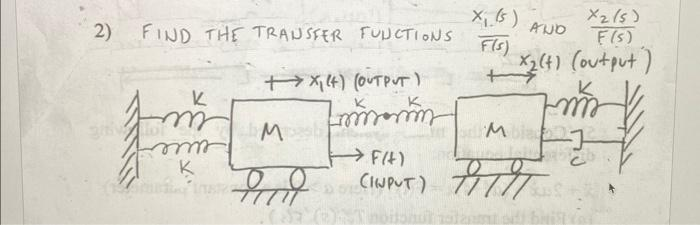Home / Expert Answers / Mechanical Engineering / 2-find-the-transfer-functions-f-s-xi-s-and-f-s-x2-s-pa888

# (Solved): 2) FIND THE TRANSFER FUNCTIONS F(s)xi(s) AND F(s)x2(s) ...2) FIND THE TRANSFER FUNCTIONS AND

We have an Answer from Expert

To find the transfer fnctino, we need to wrtie all the components of forces on both the blocks and then take the laplace transform.

1. On body 1

On taking the laplace transform,

laplace transform of the acceleration is s^2X1(s) the assumpotion is the initial conditions were zero.

We have an Answer from Expert